Get instant live expert help with Excel or Google Sheets“My Excelchat expert helped me in less than 20 minutes, saving me what would have been 5 hours of work!”

#### Post your problem and you’ll get Expert help in seconds.

Your message must be at least 40 characters
Our professional Expert are available now. Your privacy is guaranteed.

# Sum by Week Number

Excel allows us to sum the values based on the week number by using SUMIFS and WEEKNUM functions. This step by step tutorial will assist all levels of Excel users in summarizing the values based on the multiple criteria, including week number.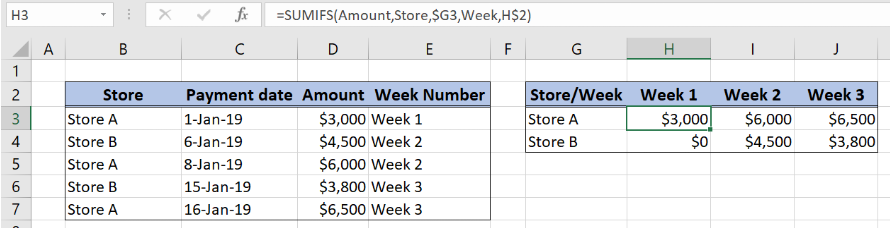Figure 1. Sum Amount by Week Number

## Syntax of the SUMIFS Formula

`=SUMIFS(sum_range, criteria_range1, criteria1, criteria_range2, criteria2)`

The parameters of the SUMIFS function are:

• sum_range – a range with values which we want to sum
• criteria_range1 – a range where we want to set our first condition
• criteria1 – the first condition for summing the values
• criteria_range2 – a range where we want to set our second condition
• criteria2 – the second condition for summing the values

## Syntax of the WEEKNUM Formula

`=WEEKNUM(date, [return_type])`

The parameters of the WEEKNUM function are:

• date – a date from which we want to get week number
• return_type – a day the weeks begins (is set to 1 by default)

## Setting up Our Data for Summing by Week Number

Our first table consists of 4 columns: “Store” (column B), “Payment date” (column C), “Amount” (column D) and “Week Number” (column E). The second one has week columns (Week 1, Week 2 and Week 3) and rows with “Store A” and “Store B”. The idea is to summarize the “Amount” values from the column “Amount” based on the Store and Week number.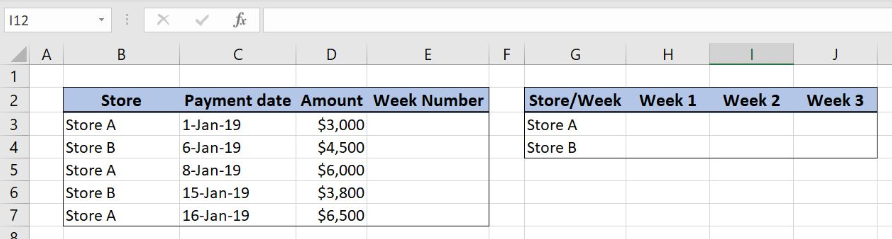Figure 2. Table structure for summarizing the “Amount” values by week number

## SUM by Week Number Using SUMIFS and WEEKNUM Functions

We want to get the total amount from column D based on the week number and store. Date format in column “Payment date” is not matchable with weeks in the second table. We will have to convert the dates to week numbers with WEEKNUM function and ampersand “&”.

The formula looks like:

`="Week "&WEEKNUM(C3,1)`

The date in the WEEKNUM function is the date in the cell C3, while the return_type is 2. The week number is linked to the string “Week” with an ampersand “&”. Formula result is now matchable with weeks from the second table.

To apply the WEEKNUM function we need to follow these steps:

• Select cell E3 and click on it
• Insert the formula: `="Week "&WEEKNUM(C3,1)`
• Press enter
• Drag the formula down to the other cells in the column by clicking and dragging the little “+” icon at the bottom-right of the cell.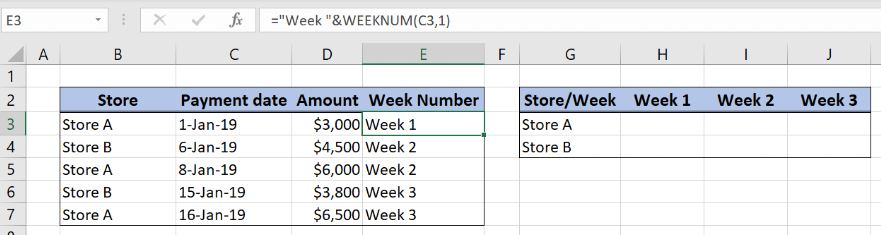Figure 3. Get the matchable week format from “Payment date”

In order to make the SUMIFS formula more clear, we will create a named range Store for cell range B3:B7, Amount for cell range C3:C7 and Week for cell range E3:E7.

To create a named range we should follow the steps:

• Select the cell range that should be named
• Click on the name box in Excel
• Write the name for the cell range and press enter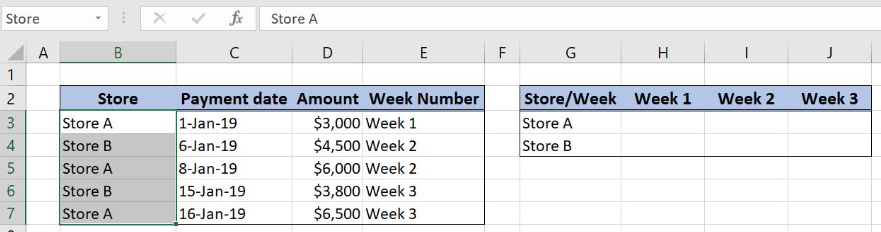Figure 4. Creating a named range Store for column “Store”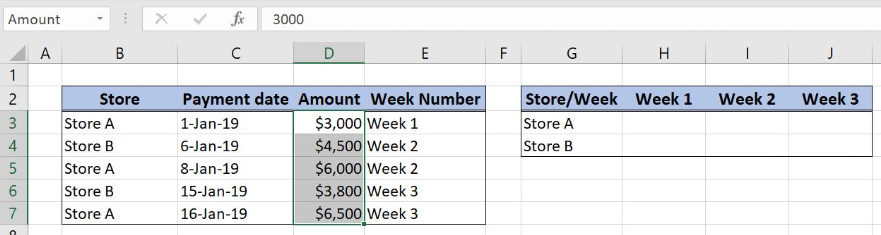Figure 5. Creating a named range Amount for column “Amount”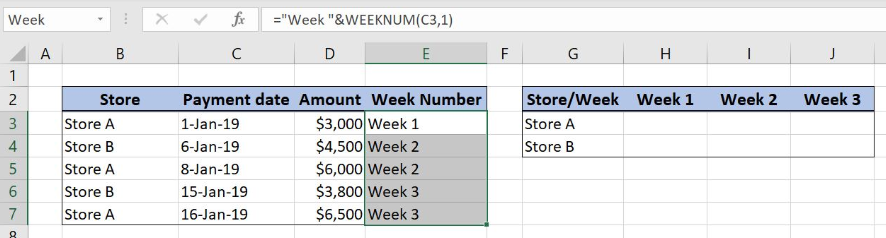Figure 6. Creating a named range Week for column “Week Number”

The formula for summarizing the values based on the week number and store looks like:

`=SUMIFS(Amount,Store,\$G3,Week,H\$2)`

The sum_range in the SUMIFS function is the named range Amount, criteria_range1 is the named range Store while the criteria1 is the cell G3 (Store A). Criteria_range2 is the named range Week while the criteria2 is the cell H2 (Week1).

To apply the SUMIFS function we need to follow these steps:

• Select cell H3 and click on it
• Insert the formula: `=SUMIFS(Amount,Store,\$G3,Week,H\$2)`
• Press enter
• Drag the formula right to the other cells in the row by clicking and dragging the little “+” icon at the bottom-right of the cell.
• Drag the formula down to the other cells in the column by clicking and dragging the little “+” icon at the bottom-right of the cell.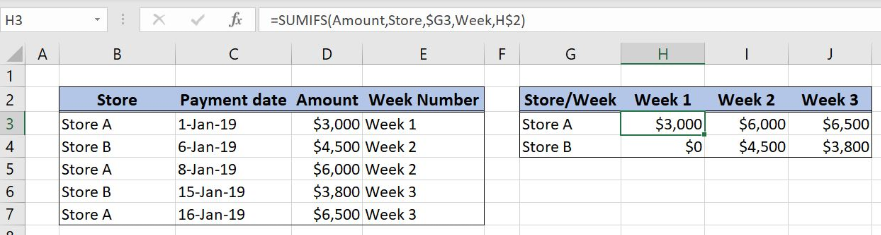Figure 7. Sum by Week number with SUMIFS and WEEKNUM functions

With SUMIFS function we can summarize the data based on the multiple criteria, store and week number. It is important to use mixed reference in order to apply formula correctly to the entire second table. In the cell \$G3 column should be locked while in the cell H\$2 row should be locked. Final formula output is the value \$3,000, a total amount in Store A for Week 1.

Most of the time, the problem you will need to solve will be more complex than a simple application of a formula or function. If you want to save hours of research and frustration, try our live Excelchat service! Our Excel Experts are available 24/7 to answer any Excel question you may have. We guarantee a connection within 30 seconds and a customized solution within 20 minutes.

### Did this post not answer your question? Get a solution from connecting with the expert.Another blog reader asked this question today on Excelchat:
Solution examplesI am looking for a formula to SUM column L, if Column A matched the relevant date and column C matches the name
Solved by Z. Y. in 16 minsIn column A I have 1500 lines of data, some of these are valued at zero, I need to write a formula which removes the values of 0 and then gives me a sum of the unique names in column b. I am aware I could filter column a to remove "0's" and then remove duplicates from the remaining cells in column B, but this must be done in a formula
Solved by O. Q. in 56 minsHi, I need some help setting up a formula that takes info from my 'Purchase Log' sheet so it updates my 'Totals By Media Channel' sheet The formula first needs to identify whether Column I reads 'Google' or 'Media Alpha' of my Purchase Log, and then I need it to SUM up everything that says 'Google' in Column I and then take that total and multiply it by 12 x 1.5 x .8 so it then updates my Totals by Media Channel sheet. I feel like it should be the 'IF' function version of this formula: =(SUM('Purchase Log'!H2:H39))*(12)*(1.5)*(0.8)
Solved by G. J. in 37 minsI need a formula that will check if 2 or more cells containing names match then add the adjacent cells to matching name together.
Solved by F. D. in 59 minsI am trying to utilize a complicated sumif or sumifs formula that can pull a total amount from a date and a drop down box from another tab.
Solved by E. B. in 15 mins## Subscribe to Excelchat.coAnother blog reader asked this question today on Excelchat: